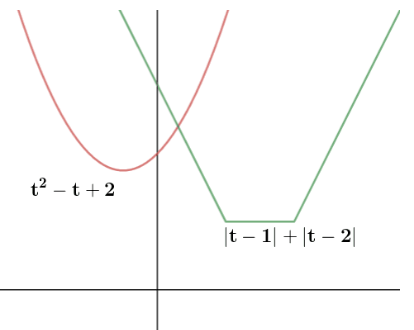Filter By

## All Questions

#### Let f be a polynomial function such thatfor allThen : Option: 1 Option: 7 Option: 13 Option: 19is given a polynomial function

Lethavingdegreebecomesdegreebecomesdegree

Now it is given thatIf we consider only degreeAssumewe getandNow Comparing coefficient ofNow Comparing coefficient ofHere a=0 or b=0

a cannot be zero so b=0

Now Comparing coefficient ofc=0

Similarly d=0

HenceandOption 2 is correct

#### The function  : N → N defined by , where N is the set of natural numbers and   denotes the greatest integer less than or equal to , is :   Option: 1 one-one and onto. Option: 2 one-one but not onto. Option: 3 onto but not one-one. Option: 4 neither one-one nor onto.

Taking x in an interval of five natural numbers, we have the following:

Therefore, here, x ∈N. hence, f(x) is neither a one-one function nor onto function.

## Crack CUET with india's "Best Teachers"

• HD Video Lectures
• Unlimited Mock Tests
• Faculty Support#### The inverse function of is Option: 1Option: 2Option: 3Option: 4Correct Option (2)

#### If  and   then  is equal to: Option: 1Option: 2Option: 3Option: 4Therefore ,

Solving the above equations,

Therefore,

Correct option (2)

## Crack NEET with "AI Coach"

• HD Video Lectures
• Unlimited Mock Tests
• Faculty SupportCorrect Option 2

#### Letbe a function defined bywheredenotes the greatest integerThen the range of f is : Option: 1Option: 2Option: 3Option: 4Piecewise function -

Greatest integer function

The function f: RR defined by f(x) = [x], xR assumes the  value of the greatest integer less than or equal to x. Such a functions called the greatest integer function.

eg;

[1.75] = 1

[2.34] = 2

[-0.9] = -1

[-4.8] = -5From the definition of [x], we

can see that

[x] = –1 for –1x < 0

[x] = 0 for 0x < 1

[x] = 1 for 1x < 2

[x] = 2 for 2x < 3 and

so on.

Properties of greatest integer function:

i) [x] ≤ x < [x] + 1

ii) x - 1 < [x] < x

iii) I ≤ x < I+1 ⇒ [x] = I where I belongs to integer.

iv) [[x]]=[x]v)

v)vi) [x] + [-x] = 2x if x belongs to integer

2[x] + 1 if x doesn’t belongs to integer

-

Domain of function, Co-domain, Range of function -

All possible values of x for f(x) to be defined is known as a domain. If a function is defined from A to B i.e. f: A?B, then all the elements of set A is called Domain of the function.

If a function is defined from A to B i.e. f: A?B, then all the elements of set B are called Co-domain of the function.

The set of all possible values of  f(x) for every x belongs to the domain is known as Range.

For example, let A = {1, 2, 3, 4, 5} and B = {1, 4, 8, 16, 25, 64, 125}. The function f : A -> B is defined by f(x) = x3. So here,

Domain : Set A

Co-Domain : Set B

Range : {1, 8, 27, 64, 125}The range can be equal to or less than codomain but cannot be greater than that.

-Correct Option (4)

## Crack JEE Main with "AI Coach"

• HD Video Lectures
• Unlimited Mock Tests
• Faculty Support#### Let S be the set of all real roots of the equation,   Then S _____. Option: 1 is a singleton set Option: 2 is an empty set Option: 3 contains at least four elements Option: 4 contains exactly two elementsOnly one point of intersection to the right of y-axis (we need postive t as 3x > 0)

Hence, singleton set

Correct Option (1)

#### Let  If  and , then the number of elements in the smallest subset of X containing both A and B is Option: 1 29 Option: 2 45 Option: 3 32 Option: 4 16

A = {2, 4, 6, 8, 10, ....., 50}

B = {7, 14, 21, 28, 35, 42, 49}

n(A  B) = {14, 28, 42}

Smallest subset of X which has all elements of A and B is the union of A and B

And we know

## Crack CUET with india's "Best Teachers"

• HD Video Lectures
• Unlimited Mock Tests
• Faculty Support#### The sum of the roots of the equation,, is :Option: 1Option: 2Option: 3Option: 4Raise to the power of 2, take

#### Letbe a function such thatfor every, thenis equal to :Option: 1Option: 2Option: 3Option: 4....(1)

Also put

..........(2)